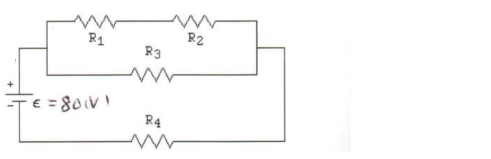# Problem: In the figure, consider the circuit sketched. The battery has emf ε = 80 volts and negligible internal resistance. The four resistors have resistances of R1 = 14 Ω, R2 = 21 Ω, R3 = 21 Ω, and R4 = 14 Ω. Find the voltage drop across R4.

###### FREE Expert Solution
91% (456 ratings)
###### Problem Details

In the figure, consider the circuit sketched. The battery has emf ε = 80 volts and negligible internal resistance. The four resistors have resistances of R1 = 14 Ω, R2 = 21 Ω, R3 = 21 Ω, and R4 = 14 Ω. Find the voltage drop across R4Frequently Asked Questions

What scientific concept do you need to know in order to solve this problem?

Our tutors have indicated that to solve this problem you will need to apply the Combining Resistors in Series & Parallel concept. You can view video lessons to learn Combining Resistors in Series & Parallel. Or if you need more Combining Resistors in Series & Parallel practice, you can also practice Combining Resistors in Series & Parallel practice problems.

How long does this problem take to solve?

Our expert Physics tutor, Douglas took 7 minutes and 38 seconds to solve this problem. You can follow their steps in the video explanation above.

What professor is this problem relevant for?

Based on our data, we think this problem is relevant for Professor Kang's class at UCF.